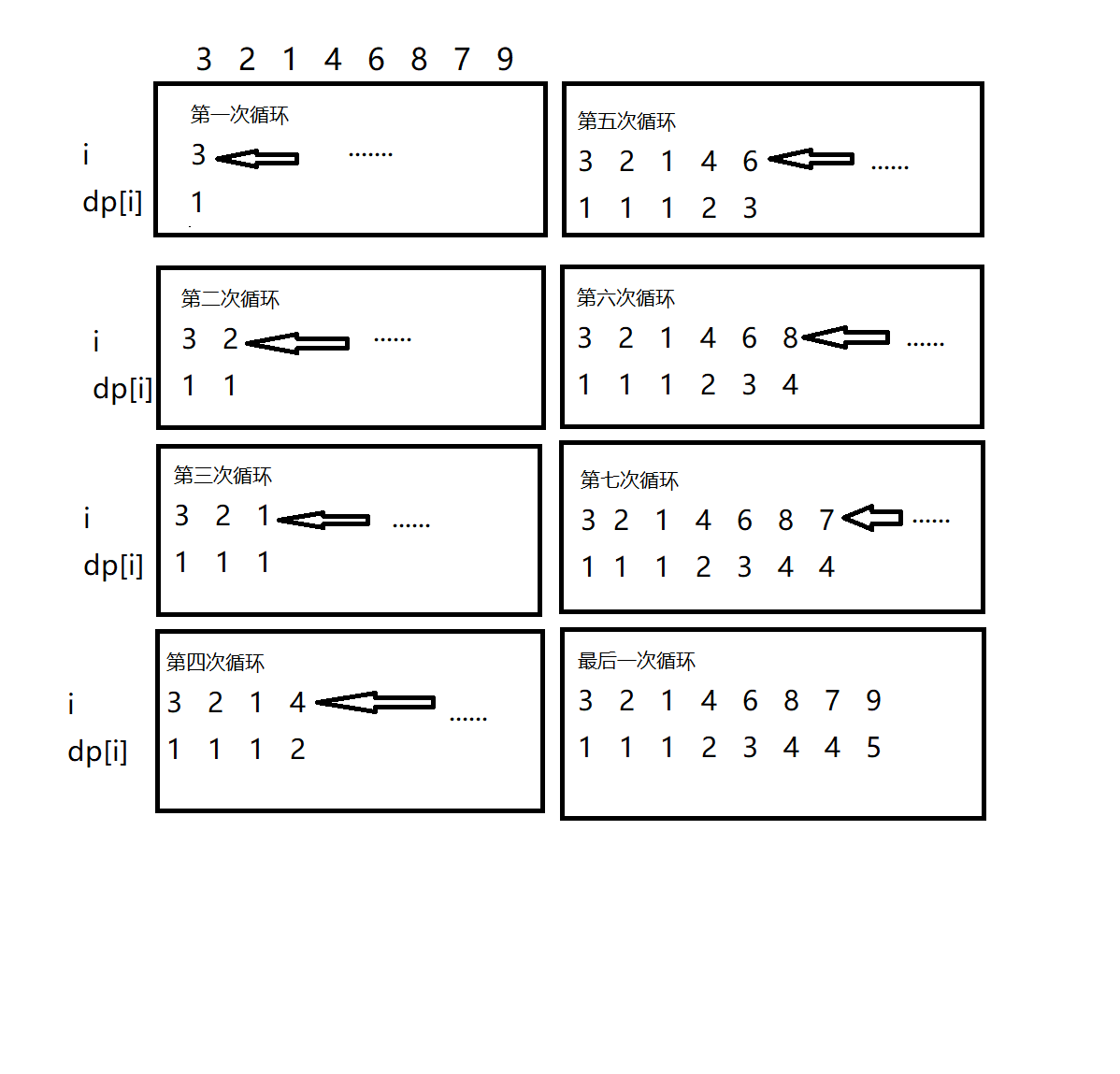#0
0
01. 云栖社区>
2. 博客>
3. 正文

## 动态规划——线性DP.1DP是D****** P*******的缩写。

so

LIS：

3   4   6   8   9

······

BUT，有的老师不会好心，会给更多限制条件，使Ans只有一种情况，那就更有难度了。

LIS问题要用动态规划做。code:

``` 1 int n,a/*用来存序列*/,dp/*dp值*/;//数组大小根据题目而定。
2 cin>>n;
3 dp=1;                                   //1的dp值为1
4 for(int i=1;i<=n;i++)
5     cin>>a[i];
6 for(int i=1;i<=n;i++)
7 {
8     for(int j=1;j<i;j++)
9     {
10         if(a[i]>a[j])
11         {
12             dp[i]=max(dp[i],dp[j]+1);      //状态转移
13         }
14     }
15 }
16 cout<<dp[n]<<endl;```

注意要初始化dp [ 1 ] = 1.剩下的为 0.

2   1   5   3   6   4   6   3

code：

``` 1 const int maxn=1e5+5;
2 int a[maxn];
3 int n;
4 int dp[maxn];
5 int ans=1;
6 int find(int x){
7     int l=1,r=ans,m;
8     while(l<r){
9         m=l+(r-l)/2;
10         if(dp[m]>=a[x]){
11             r=m;
12         }
13         else{
14             l=m+1;
15         }
16     }
17     return l;
18 }//二分查找
19 int main(){
20     scanf("%d",&n);
21     for(int i=1;i<=n;i++)scanf("%d",&a[i]);
22     dp=a;
23     for(int i=2;i<=n;i++){
24         if(a[i]>dp[ans]){
25             dp[++ans]=a[i];
26         }
27         else{
28             int pos=find(i);
29             dp[pos]=a[i];
30         }
31     }
32     printf("%d",ans);
33     return 0;
34 }```

+ 关注